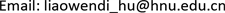SGSmart Grid2161-8763Scientific Research Publishing10.12677/SG.2018.86061SG-27894SG20180600000_79633525.pdf工程技术 全直流供电建筑储能最优运行策略研究 Research on Optimal Operation Strategy of All-DC Power Supply for the Building Energy Storage 闻迪21雨桐2121文宇21深圳市建筑科学研究院股份有限公司，广东 深圳null301020180806555564© Copyright 2014 by authors and Scientific Research Publishing Inc. 2014This work is licensed under the Creative Commons Attribution International License (CC BY). http://creativecommons.org/licenses/by/4.0/Copyright © 2018 by authors and Hans Publishers Inc.1. 引言

2. 全直流建筑供电模型

1) 电网取电：可取功率为DC2 × 200 kW；

2) 光伏发电：最大取电功率约100 kWp；

1) 集中式储能，布置于集中储能柜内，约100 kWh；

2) 分布式储能：分别布置于空调、房间等空间内，有效储能量约30 kWh；

3. 优化目标与优化方式

W = [ w 1 , w 2 , ⋯ , w 24 ] (1)

V = [ v 1 , v 2 , ⋯ , v 24 ] (2)

B = [ b 1 , b 2 , ⋯ , b 24 ] (3)

P = [ p 1 , p 2 , ⋯ , p 24 ] (4)

P = W − V − B (5)

{ ∑ i = 1 24 b i = 0 0 ≤ b j ≤ Q S O C = Q − ∑ i = 1 j b i Q × 100 S O C ∈ ( 0 , 100 ] (6)

F 1 ( P ) = ∑ i = 1 24 ( p i − p ¯ ) 2 (7)

p ¯ = ∑ i = 1 24 p i 24 (8)

min   F 1 ( P ) = ∑ i = 1 24 ( p i − p ¯ ) 2 (9)

min   F 2 ( P ) = ∑ i = 1 23 ( p i − p i − 1 ) (10)

M = [ m 1 , m 2 , ⋯ , m 24 ] (11)

min   F 3 ( P ) = ∑ i = 1 24 p i × m i (12)

G = [ g 1 , g 2 , ⋯ , g 24 ] (13)

min   F 4 ( P ) = ∑ i = 1 24 | p i − g i | (14)

4. 储能优化策略与控制对象

p s e t = p ¯ (15)

2) 储能电池容量≥充放电周期内超出均值能量的累积值:

Q ≥ ∑ i = 1 24 ( p i + − p ¯ ) (16)

1) 比例充放，即以小时耗电量与小时耗电量成比例补偿：

b k = 2 Q ( p k − p ¯ ) ∑ j = 1 24 | p ¯ − p j | (18)

2) 平均充放，即每小时放电/充电量相同进行补偿：

{ b ¯ + = Q l e n g t h ( p k > p ¯ ) b ¯ − = Q l e n g t h ( p k < p ¯ ) (19)

3) 峰值充放，即只在用电峰值3小时与用电谷值5小时进行放电/充电补偿，其余时间储能电池不充不放。

5. 优化仿真

Modeling and simulation parameter

P 0 冬 = W 冬 − V 冬 = [ 37.85 , 75.21 , 55.05 , 45.88 , 48.58 , 56.14 , 64.58 , 47.19 , 57.38 , 69.70 , 48.93 ,                     26.87 , 25.33 , 22.62 , 19.33 , 8.55 , 6.70 , 3.03 , 3.03 , 3.03 , 3.03 , 3.03 , 3.03 , 15.40 ] P 0 夏 = W 夏 − V 夏 = [ 66.83 , 70.33 , 113.40 , 98.48 , 78.62 , 74.79 , 67.06 , 69.79 , 61.02 , 73.75 , 93.94 ,                     78.45 , 42.11 , 46.36 , 45.73 , 44.61 , 8.64 , 6.78 , 3.12 , 3.12 , 3.12 , 3.12 , 3.12 , 2.49 ]

3 kinds of energy storage and charging optimization strategy evaluation valu

6. 结论